# Surface Area Calculator

Surface Area Calculator The surface area of a solid is a measure of the total area occupied by the surface of an object. All of the objects addressed in this calculator are described in more detail on the Volume Calculator and Area Calculator pages.

### What is the formula for the surface area?

We can also label the length (l), width (w), and height (h) of the prism and use the formula, SA=2lw+2lh+2hw, to find the surface area.

### Sphere Surface Area Calculator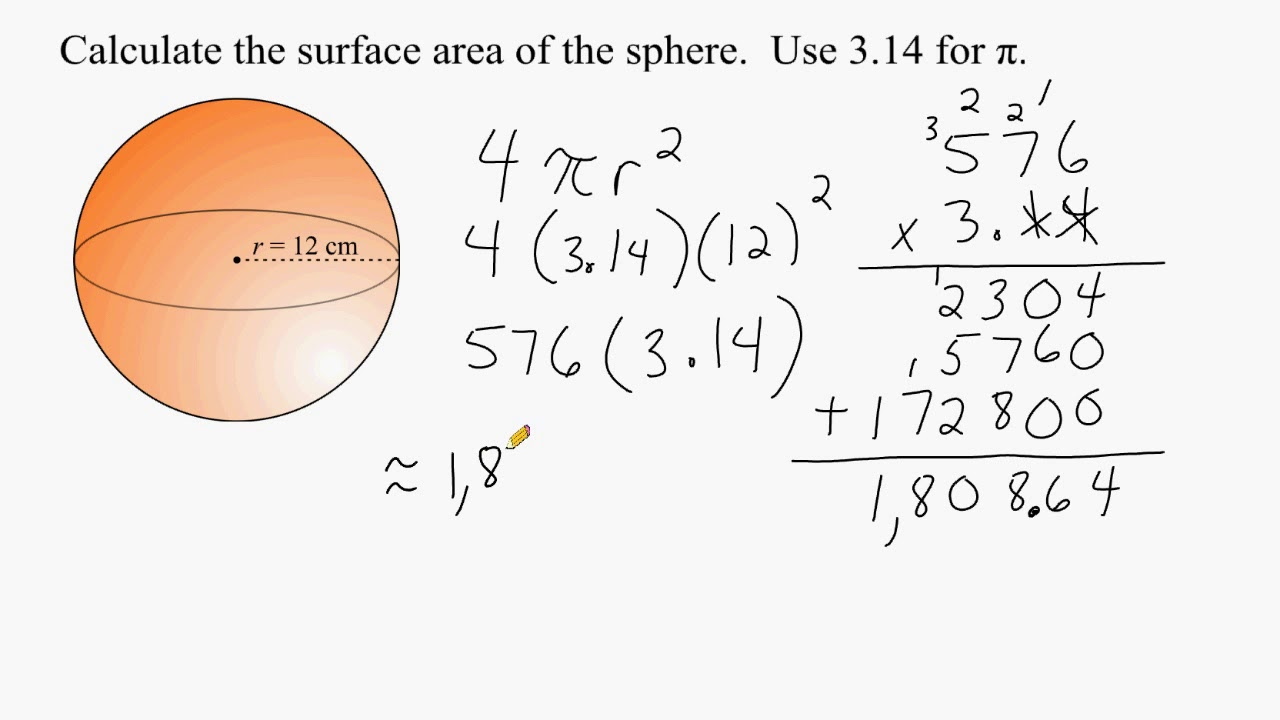SA = 4πr2
where r is the radius

SA = 4 × π × 0.3252 = 1.327 in2

### Cone Surface Area Calculator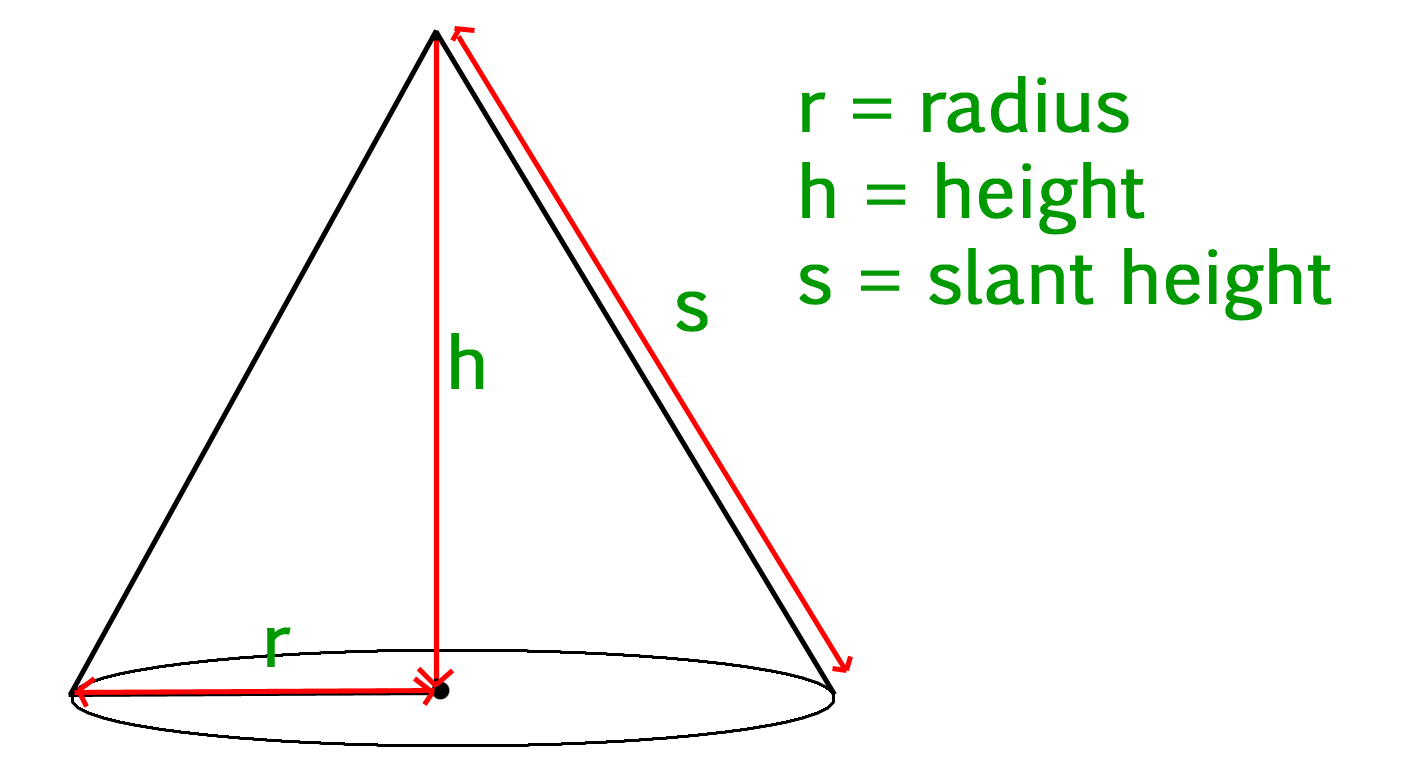base SA = πr2
lateral SA = πr√r2 + h2
total SA = πr(r + √r2 + h2)
where r is radius and h is height

lateral SA = π × 0.4√0.42 + 0.52 = 0.805 ft2

### Cube Surface Area CalculatorSA = 6a2
where a is edge length

SA = 6 × 42 = 96 in2

### Cylindrical Tank Surface Area Calculator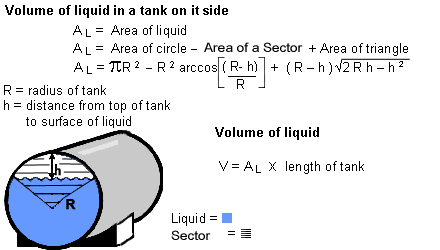base SA = 2πr2
lateral SA = 2πrh
total SA = 2πr(r + h) where r is radius and h is height

total SA = 2π × 3.5(3.5 + 5.5) = 197.920 ft2

### Rectangular TankSA = 2lw + 2lh + 2wh
where l is length, w is width, and h is height

SA = (2 × 3 × 4) + (2 × 4 × 5) + (2 × 3 × 5) = 94 ft2

### Capsule Surface Area CalculatorSA = 4πr2 + 2πrh
where r is radius and h is height

SA = 4π × 0.052 + 2π × 0.05 × 0.5 = 0.188 in2

### Spherical Cap Surface Area Calculatorspherical cap SA = 2πRh
base SA = πr2
Total solid sphere SA = 2πRh + πr2
where R is spherical cap radius, r is base radius, and h is height

SA = 2π × 0.80 × 0.53 = 2.664 ft2

### Conical Frustum Surface Area Calculator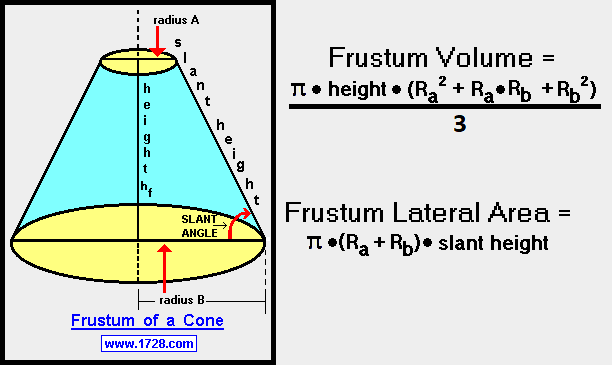circular end SA = π(R2 + r2)
lateral SA = π(R+r)√(R-r)2 + h2
total SA = π(R2 + r2) + π(R+r)√(R-r)2 + h2
where R and r are radii of the ends, h is the height

total SA = π(12 + 0.32) + π(1 + 0.3) √(1 – 0.3)2 + 1.52 = 10.185 ft2

### Ellipsoid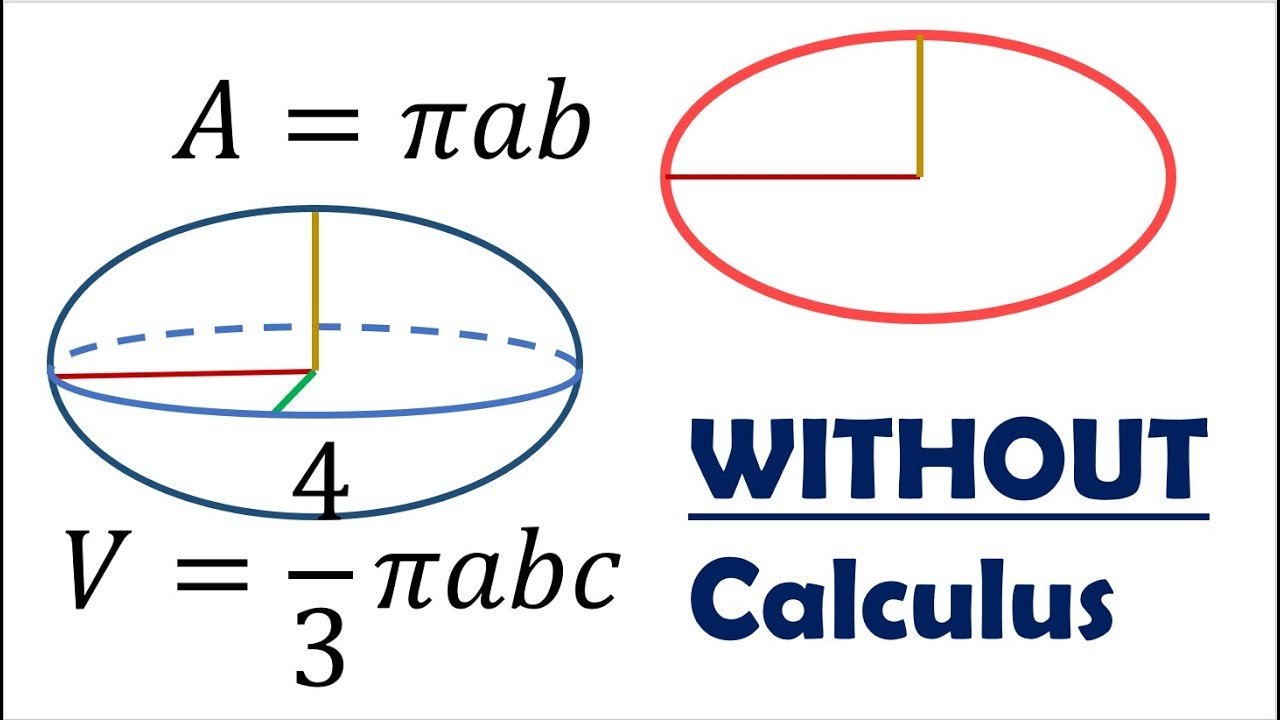SA ≈ 4π 1.6√(a1.6b1.6 + a1.6c1.6 + b1.6c1.6)/3
where ab, and c are the axes of the ellipse

SA ≈ 4π 1.6√(0.11.60.21.6 + 0.11.60.351.6 + 0.21.60.351.6)/3 = 0.562 in2

### Square Pyramid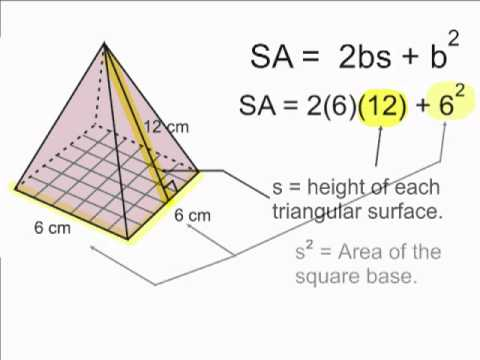base SA = a2
lateral SA = 2a√(a/2)2 + h2
total SA = a2 + 2a√(a/2)2 + h2

total SA = 32 + 2 × 3√(3/2)2 + 52 = 40.321 ft2

### Common Area Units

 Unit meter2 kilometer2 1,000,000 centimeter2 0.0001 millimeter2 0.000001 micrometer2 0.000000000001 hectare 10,000 mile2 2,589,990 yard2 0.83613 foot2 0.092903 inch2 0.000645

MG to ML

CC

### What is normal body surface area?

The “normal” body surface area is generally taken to be 1.7 m2 but, in actual fact, the body surface area depends on more than just height and weight. Other influential factors include the age and gender of the individual. For example: Average body surface area for adult men: 1.9 m2